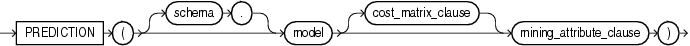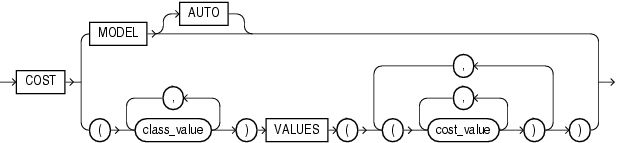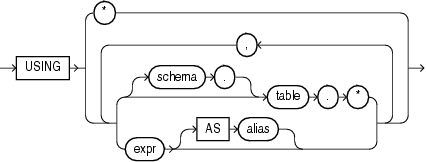# PREDICTION

SyntaxDescription of the illustration prediction.gif

cost_matrix_clause::=Description of the illustration cost_matrix_clause.gif

mining_attribute_clause::=Description of the illustration mining_attribute_clause.gif

Purpose

This function is for use with mining models created by the `DBMS_DATA_MINING` package or with Oracle Data Miner. It returns the best prediction for the model. The data type returned depends on the target value type used during the build of the model. For regression models, this function returns the expected value.

cost_matrix_clause  The `COST` clause is relevant for all classification models.

• Specify `COST` `MODEL` to indicate that the scoring should be performed by taking into account the scoring cost matrix associated with the model. If no such scoring cost matrix exists, then the database returns an error.

• Specify `COST` `MODEL` `AUTO` if the existence of a cost matrix is unknown. In this case:

• If the stored cost matrix exists, then the function returns the lowest cost prediction using the stored cost matrix.

• If no stored cost matrix exists, then the function returns the highest probability prediction.

• Use the `VALUES` clause (the bottom branch of the `cost_matrix_clause`) to specify an inline cost matrix. You can use an inline cost matrix regardless of whether the model has an associated scoring cost matrix. Refer to Oracle Data Mining Application Developer's Guide for an example of an inline cost matrix

If you omit the `cost_matrix_clause` clause, then the best prediction is the target class with the highest probability. If two or more classes are tied with the highest probability, the database chooses one class.

mining_attribute_clause This maps the predictors that were provided when the model was built. Specifying `USING *` maps to all to the columns and expressions that can be retrieved from the underlying inputs (tables, views, and so on).

• If you specify more predictors in the `mining_attribute_clause` than there are predictors used by the model, then the extra expressions are silently ignored.

• If you specify fewer predictors than are used during the build, then the operation proceeds with the subset of predictors you specify and returns information on a best-effort basis. All types of models will return a result regardless of the number of predictors you specify in this clause.

• If you specify a predictor with the same name as was used during the build but a different data type, then the database implicitly converts to produce a predictor value of the same type as the original build.

Example

The following example returns by gender the average age of customers who are likely to use an affinity card. The `PREDICTION` function takes into account only the `cust_marital_status`, `education`, and `household_size` predictors.

This example, and the prerequisite data mining operations, including the creation of the view, can be found in the demo file `\$ORACLE_HOME/rdbms/demo/dmdtdemo.sql`. General information on data mining demo files is available in Oracle Data Mining Administrator's Guide. The example is presented here to illustrate the syntactic use of the function.

```SELECT cust_gender, COUNT(*) AS cnt, ROUND(AVG(age)) AS avg_age
FROM mining_data_apply_v
WHERE PREDICTION(DT_SH_Clas_sample COST MODEL
USING cust_marital_status, education, household_size) = 1
GROUP BY cust_gender
ORDER BY cust_gender;

C        CNT    AVG_AGE
- ---------- ----------
F        170         38
M        685         42
```